# Introduction to K-Nearest Neighbor (KNN)KNN or k-nearest neighbor is a supervised learning algorithm. KNN is also known as an Instance-based Learning Algorithm. KNN is a technique of identifying the category or class label of any particular point in sample space based on its nearest samples. The letter k in KNN denotes how many samples we will consider in the neighborhood to predict the class label of our concerned point in sample space.

### Introduction

KNN or k-nearest neighbor is a supervised learning algorithm. It can be applied to both Regression and Classification problem-solving. KNN is a technique of identifying the category or class label of any particular point in sample space based on its nearest samples. The letter k in KNN denotes how many samples we will consider in the neighborhood to predict the class label of our concerned point in sample space.

KNN is also known as an Instance-based Learning Algorithm. In Instance-based learning when we get the training samples we don't process them and learn a model instead we store the training samples and when we need to classify the instance then we do the training and class-label association with the test samples. So the Instance-based Learning Algorithm is also called a Lazy Algorithm.

### KNN in Classification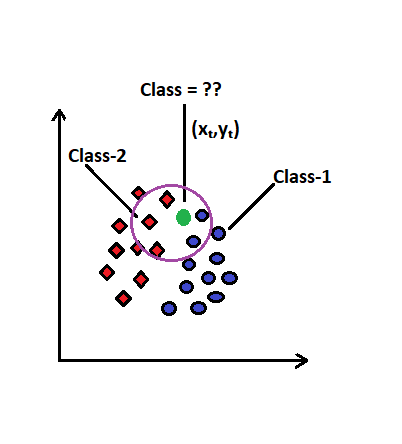Image by Author

We are given different sample points (x₁,y₁),(x₂,y₂),(x₃,y₃),…..,(xₙ,yₙ). All these points are categorized either in class label-1 or class label-2. We introduce a point (xₜ,yₜ)and want to predict its class label. So we use the KNN approach and follow the below steps.

Step 1: Choose the value of k

We can decide the value of k which will determine the total number of nearest samples we need to consider to predict the value of the test sample(xₜ,yₜ). Here in our case, we consider k=5.

Step 2: Calculate the distance

Determine the 5 nearest samples w.r.t test sample using the Euclidean distance formula. The Euclidean distance is calculated using the below formula for any two given points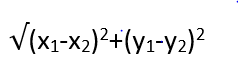Image by Author

Step 3: Classify the nearest points based on Category

It can be observed from the figure that out of 5 nearest points 3 of them belong to class label-2 and two of them belong to a class label-1. So going by the majority it can be concluded that point (xₜ,yₜ) belongs to a class label-2.

### KNN in Regression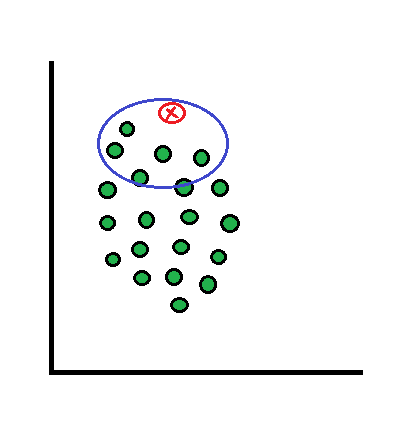Image by Author

In the case of KNN in Regression, we will be using almost the same approach as Classification. There will be a difference in only step-3 where we won't be taking the majority class label for the test sample. Instead, we will be taking the average of all the class labels and set the value as a class label for the test sample. E.g. in the above image, we have considered k=5 to determine the class label for the test sample. By using the distance formula we identify the 5 nearest training sample points and identify their class labels. As it is a regression problem the class-label for all the 5 training samples is similar. So taking the average of all the test samples’ class labels gives us the class label of the test sample.

### Attributes and Weights

We saw that every sample point has its own x and y coordinates. But apart from the coordinates, there are features or attributes which represent the points in the sample space. These attributes also highly contribute to separate the points into various class labels. So the Euclidean distance must be calculated from all those attributes as well while finding the nearest k-points.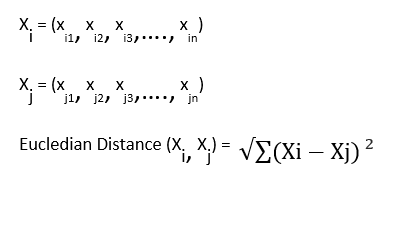Image by Author

Now we know that we consider the average value of class labels for the Regression KNN problem. When we consider the large values of k then it becomes necessary to take the average of class labels rather than majority consideration during classification. Below are some of the reasons :

1. noise in attributes- due to the presence of noise the nearest sample point to the test sample may not be able to capture all the characteristics of the test sample and rather maybe the slightly farther away point will be able to capture.
2. noise in class labels- Due to the presence of noise in class labels there is high chances of misclassification of test samples
3. partially Overlapping class labels- overlapping of class labels result in the incapability of the algorithm in allocating the correct class labels of sample test points.

Have you ever thought about why are we not considering the weight of the attributes? If the weights are considered equal for all attributes then the below assumptions will be true about the sample space:

1. All the attributes have the same scale. Possessing the same scale means that all the attributes are measured using the same unit i.e. measuring height measurement of students in cm and feet is irrelevant.
2. The range of all attributes must be the same. E.g. the values of all the attributes must vary from 0 to 100 and not from 0 to 1000.

### Limitations

Imbalance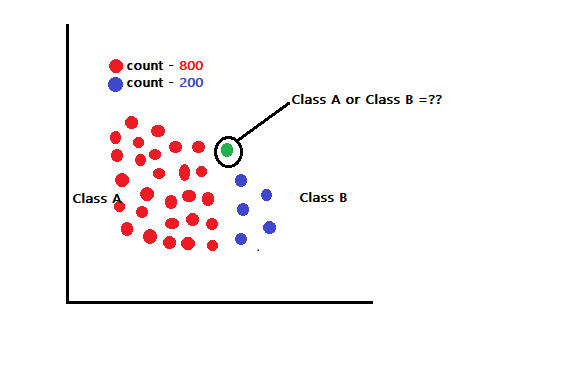Image by Author

Consider a case in a sample space where there are around 1000 points either classified as class A or class B. Assume that out of 1000 points 800 points belong to class-A which indicates that the dataset is highly imbalanced. Can this impact the classification of new test samples? Yes, definitely !! Consider that we want to find the class label of point X in the sample space. If we consider the k value to be very large say around 150 then the imbalanced dataset will push point X to fall into class-A forcefully which might result in misclassification.

Outlier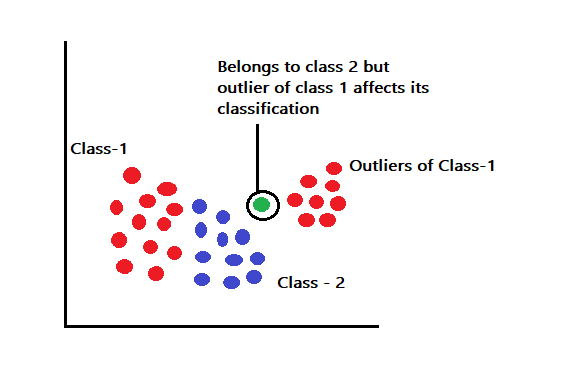Image by Author

Consider the above image having outliers belonging to class -1. Assume that we want to predict a point in between the class-1 labeled outliers and class-2 labeled training points. The test point might belong to the class-2 label but due to the presence of a class-1 outlier, the points might just be misclassified wrongly in class-1.

So the limitation of KNN is that due to the presence of an Imbalanced or Outlier dataset it might misclassify the points.

## Most popular Data Science and Machine Learning courses — July 2020

Most popular Data Science and Machine Learning courses — August 2020. This list was last updated in August 2020 — and will be updated regularly so as to keep it relevant

## Pipelines in Machine Learning | Data Science | Machine Learning | Python

Machine Learning Pipelines performs a complete workflow with an ordered sequence of the process involved in a Machine Learning task. The Pipelines can also

## Data Science Projects | Data Science | Machine Learning | Python

Practice your skills in Data Science with Python, by learning and then trying all these hands-on, interactive projects, that I have posted for you.

## Data Science Projects | Data Science | Machine Learning | Python

Practice your skills in Data Science with Python, by learning and then trying all these hands-on, interactive projects, that I have posted for you.

## Data Science Projects | Data Science | Machine Learning | Python

Practice your skills in Data Science with Python, by learning and then trying all these hands-on, interactive projects, that I have posted for you.